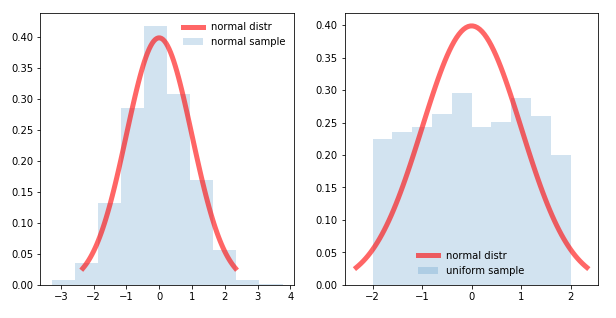### Why does Outlier Detection Need AI?An AI based approach increases accuracy and can even make the impossible possible.

What is an Outlier?

Put simply, an outlier is a piece of data or observation that differs drastically from a given norm.

In the image above, the red fish is an outlier. Clearly differing by color, but also by size, shape, and more obviously direction. As such, the analysis of detecting outliers in data fall into two categories: univariate, and multivariate
• Univariate: considering a single variable
• Multivariate: considering multiple variables

Outlier Detection in Industrial IoT

In Industrial IoT use cases, outlier detection can be instrumental in specific use cases such as understanding the health of your machine. Instead of looking at characteristics of a fish like above, we are looking at characteristics of a machine via data such as sensor readings.

The goal is to learn what normal operation looks like where outliers are abnormal activity indicative of a future problem.

Statistical Approach to Outlier DetectionStatistical/probability based approaches date back centuries. You may recall back the bell curve. The values of your dataset plot to a distribution. In simplest terms, you calculate the mean and standard deviation of that distribution. You then can plot the location of x standard deviations from the mean and anything that falls beyond that is an outlier.

A simple example to explore using this approach is outside air temperature. Looking at the low temperature in Boston for the month of January from 2008-2018 we find an average temperature of ~23 degrees F with a standard deviation of ~9.62 degrees. Plotting out 2 standard deviations results in the following.

Interpreting the chart above, any temperature above the gray line or below the yellow can be considered outside the range of normal...or an outlier.

Why do we need AI?
If we just showed that you can determine outliers using simple statistics, then why do we need AI at all? The answer depends on the type of outlier analysis.

Why AI for Univariate Analysis?
In the example above, we successfully analyzed outliers in weather looking at a single variable: temperature.

So, why should we complicate things by introducing AI to the equation? The answer has to do with the distribution of your data. You can run univariate analysis using statistical measures, but in order for the results to be accurate, it is assumed that the distribution of your data is "normal". In other words, it needs to fit to the shape of a bell curve (like the left image below).

However, in the real world, and specifically in industrial use cases, the resulting sensor data is not perfectly normal (like the right image below).As a result, statistical analysis on a non-normal dataset would result in more false positives and false negatives.

The Need for AI
AI-based methods on the other hand, do not require a normal distribution and finds patterns in the data that result in much higher accuracy. In the case of the weather in Boston, getting the forecast slightly wrong does not have a huge impact. However, in industries such as rail, oil and gas, and industrial equipment, trust in the accuracy of your results has a long lasting impact. An impact that can only be achieved by AI.

Why AI for Multivariate Analysis?
The case for AI in a multivariate analysis is a bit more straight forward. Effectively, when we are looking at a single variable we can easily plot the results on a plane such as the temperature chart or the normal and non-normal distribution charts above.

However, if we are analyzing multiple points, such as the current, voltage and wattage of a motor, or vibration over 3 axis, or the return temp and discharge temp of an HVAC system, plotting and analyzing with statistics has its limitations. Just visualizing the plot becomes impossible for a human as we go from a single plane to hyperplanes as shown below.The Need for AI
For multivariate analysis, visual inspection starts to go beyond human capabilities while technical analysis goes beyond statistical capabilities. Instead, AI can be utilized to find patterns in the underlying data in order to learn normal operation and adequately monitor for outliers. In other words, for multivariate analysis AI starts to make the impossible possible.

Summary
Statistics and probability has been around far longer than anyone reading this post. However, not all data is created equal and in the world of industrial IoT, statistical techniques have crucial limitations.

AI-based techniques go beyond these limitations helping to reduce false positives/negatives and often times making robust analysis possible for the first time.

At Elipsa, we build simple, fast and flexible AI for IoT. Get free access to our Community Edition to start integrating machine learning into your applications.Elipsa AI that is simple, fast, and flexible enabling IoT developers to integrate AI into their applications